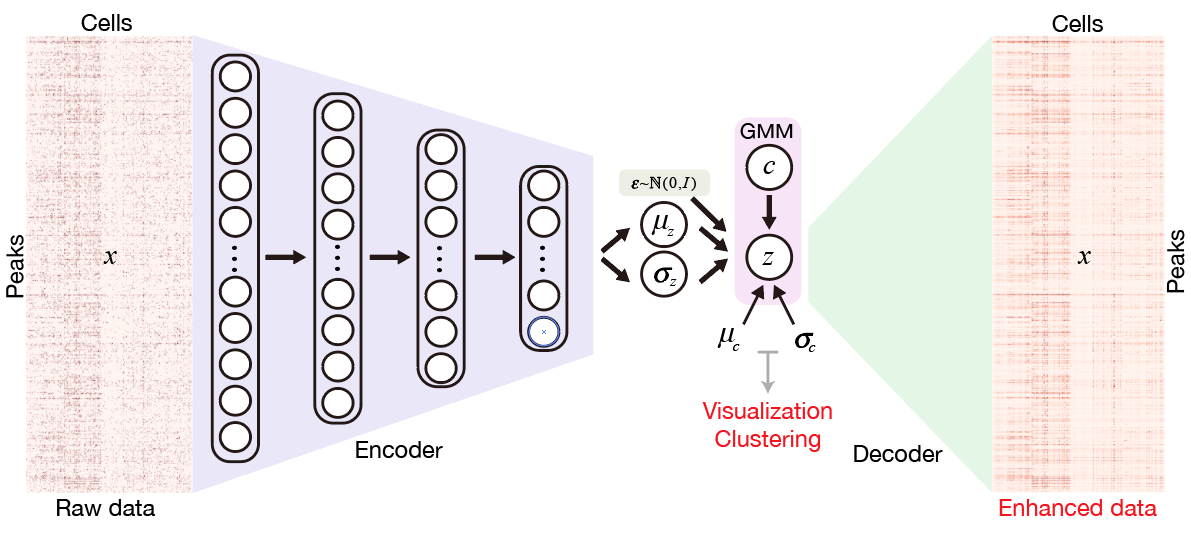# yejg2017 / scATAC_DeepLearning

Single-cell ATAC-seq analysis via Latent feature Extraction

Geek Repo

Github PK Tool

# Single-Cell ATAC-seq analysis via Latent feature Extraction## Installation

SCALE neural network is implemented in Pytorch framework.
Running SCALE on CUDA is recommended if available.

#### install from GitHub

``````git clone git://github.com/jsxlei/SCALE.git
cd SCALE
python setup.py install
``````

Installation only requires a few minutes.

## Quick Start

#### Input

• either a count matrix file:
• row is peak and column is barcode, in txt / tsv (sep="\t") or csv (sep=",") format
• or a folder contains three files:
• count file: count in mtx format, filename contains key word "count" / "matrix"
• peak file: 1-column of peaks chr_start_end, filename contains key word "peak"
• barcode file: 1-column of barcodes, filename contains key word "barcode"

#### Run

with known cluster number k:

``````SCALE.py -d [input] -k [k]
``````

with estimated cluster number k by SCALE if k is unknown:

``````SCALE.py -d [input]
``````

#### Output

Output will be saved in the output folder including:

• model.pt: saved model to reproduce results cooperated with option --pretrain
• feature.txt: latent feature representations of each cell used for clustering or visualization
• cluster_assignments.txt: clustering assignments of each cell
• tsne.txt: 2d t-SNE embeddings of each cell
• tsne.pdf: visualization of 2d t-SNE embeddings of each cell

#### Imputation

Get binary imputed data in folder binary_imputed with option --binary (recommended for saving storage)

``````SCALE.py -d [input] --binary
``````

or get numerical imputed data in file imputed_data.txt with option --impute

``````SCALE.py -d [input] --impute
``````

#### Useful options

• save results in a specific folder: [-o] or [--outdir]
• filter rare peaks if the peaks quality if not good or too many, default is 0.01: [-x]
• filter low quality cells by valid peaks number, default 100: [--min_peaks]
• modify the initial learning rate, default is 0.002: [--lr]
• change the batch size, default is 32: [--batch_size]
• change iterations by watching the convergence of loss, default is 30000: [-i] or [--max_iter]
• change random seed for parameter initialization, default is 18: [--seed]
• binarize the imputation values: [--binary]

#### Note

If come across the nan loss,

• try another random seed
• filter peaks with harsher threshold like -x 0.04 or -x 0.06
• change the initial learning rate to 0.0002

#### Help

Look for more usage of SCALE

``````SCALE.py --help
``````

Use functions in SCALE packages.

``````import scale
from scale import *
from scale.plot import *
from scale.utils import *
``````

## Tutorial

Tutorial Forebrain Run SCALE on dense matrix Forebrain dataset (k=8, 2088 cells)

Tutorial Mouse Atlas Run SCALE on sparse matrix Mouse Atlas dataset (k=30, ~80,000 cells)